# How to Handle Pivot Tables using GETPIVOTDATA in Google Sheets

Learn how to use GETPIVOTDATA, a relatively new function in Google Sheets, to copy data from a Pivot Table to another cell.1. Get the sum of a column
2. Get the value of an item in a 1-tier row label
3. Get the value of an item in a 2-tier row label
4. Get the value using the choices stored in other cells
5. Get the value using the choices stored in a drop-down box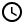8 Minutes

Pivot Table is a powerful feature in Google Sheets that helps you summarize large amounts of data stored in your sheets. To review how to use Pivot Table in Google Sheets, you can check the following tutorials:

In this tutorial, we will use the GETPIVOTDATA, a relatively new function in Google Sheets, to copy data from a Pivot Table to another cell. The advantage of GETPIVOTDATA is that it can easily adjust for changes in the composition of the Pivot Table. You should definitely use GETPIVOTDATA if there are changes occurring on the source sheet all the time!

## Get the sum of a column

For this section, we have the following Pivot Table:

Our Pivot Table summarizes the sales of certain items for one week. There is the column containing row headers of sold items followed by four columns of data.

For our first example, we just want to copy the Grand Total of the “SUM of amount” column. This column is the second column in the Pivot Table. For this purpose, the syntax of the GETPIVOTDATA function is:

=GETPIVOTDATA(“name_of_column”,first_cell_of_pivotdata)

Where name_of_column is the name of the column from where you want to get the Grand Total, and the first_cell_of_pivotdata is the reference to the first cell of the Pivot Table in the upper-left corner. Any cell that is covered by the Pivot Table can be chosen as well, but it works best with the upper-leftmost cell, which is often where the Pivot Table is inserted. For our example, the exact formula is:

=GETPIVOTDATA("SUM of amount",A1)

And we can get the corresponding value:

## Get the value of an item in a 1-tier row label

We will use the same Pivot Table as in the previous section.

For this case, we now want to get the value of “SUM of amount” for a specific item, let’s say, for a 12-egg set. To do so, we need to add additional input to the formula:

=GETPIVOTDATA(“name_of_column”,first_cell_of_pivotdata, “row_label_column”, “row_label”)

Where name_of_column is the name of the column from where you want to get the specific value, the first_cell_of_pivotdata is the reference to the first cell of the Pivot Table in the upper-left corner, the row_label_column is the name of the column containing the row labels (the first column in the Pivot Table generated), and the row_label the specific row label you are looking for. For our example, that is

=GETPIVOTDATA("SUM of amount",A1,"item","12-egg set")

The result is: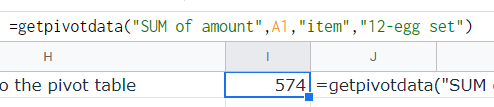GETPIVOTDATA for a specific item in a 1-tier label Pivot Table.

## Get the value of an item in a 2-tier row label

It is possible to create a Pivot Table with a 2-tier row label. A portion of it is shown below:

As you can see, this has two columns for row labels, the first column we will label as row label, and the second one we will label as row sublabel.

The formula format that we have in the previous section, shown below:

=GETPIVOTDATA(“name_of_column”,first_cell_of_pivotdata, “row_label_column”, “row_label”)

This will still work perfectly, but it will give you the subtotal for each main row label. If we want to select a specific sub-label, we need to specify it in the formula. Fortunately the syntax is not complicated:

=GETPIVOTDATA(“name_of_column”,first_cell_of_pivotdata, “row_label_column”, “row_label”, “row_sublabel_column”, “row_sublabel”)

Where:

name_of_column is the name of the column from where you want to get the specific value

first_cell_of_pivotdata is the reference to the first cell of the Pivot Table in the upper-left corner

row_label_column is the name of the column containing the row labels (the first column in the Pivot Table generated)

row_label the specific row label you are looking for

row_sublabel_column is the name of the column containing the row sublabels (the first column in the Pivot Table generated)

row_sublabel the specific row sub-label you are looking for

For example, we want to find the sum of the amount of 12-egg sets sold on July 15. The resulting formula is:

=getpivotdata("SUM of amount",A1,"item","12-egg set","date","July 15")

The result is: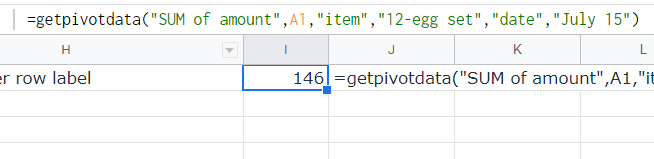GETPIVOTDATA for a specific item in a 2-tier label Pivot Table.

## Get the value using the choices stored in other cells

You can also store the name_of_column, row_label_column, row_label, row_sublabel_column, and row_sublabel to other cells and point to it in the GETPIVOTDATA function.

Below is a snippet for 1-tier label Pivot Table: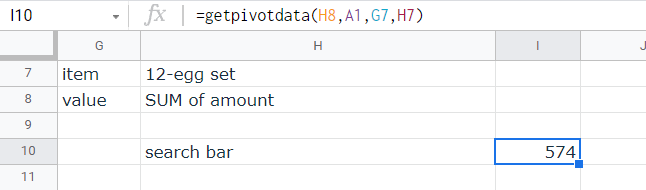GETPIVOTDATA using strings in other cells for a 1-tier Pivot Table.

Below is a snippet for 2-tier label Pivot Table: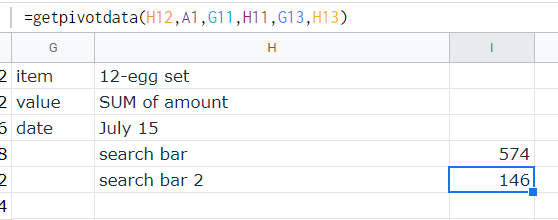GETPIVOTDATA using strings in other cells for a 2-tier Pivot Table.

## Get the value using the choices stored in a drop-down box

Finally, using the same syntax, you can design the formula to use choices from a set of drop-down boxes. Below is a snippet for a 2-tier label Pivot Table: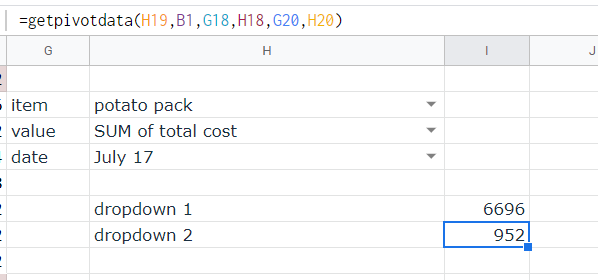GETPIVOTDATA using strings in drop-down boxes for a 1-tier Pivot Table.

## Sample sheet

To further see the workings of the GETPIVOTDATA function, check the sample sheet below:

GETPIVOTDATA sample sheet

Oops! Something went wrong while submitting the form.

December 13, 2021Pivot Table is a powerful feature in Google Sheets that helps you summarize large amounts of data stored in your sheets. To review how to use Pivot Table in Google Sheets, you can check the following tutorials:

In this tutorial, we will use the GETPIVOTDATA, a relatively new function in Google Sheets, to copy data from a Pivot Table to another cell. The advantage of GETPIVOTDATA is that it can easily adjust for changes in the composition of the Pivot Table. You should definitely use GETPIVOTDATA if there are changes occurring on the source sheet all the time!

## Get the sum of a column

For this section, we have the following Pivot Table:

Our Pivot Table summarizes the sales of certain items for one week. There is the column containing row headers of sold items followed by four columns of data.

For our first example, we just want to copy the Grand Total of the “SUM of amount” column. This column is the second column in the Pivot Table. For this purpose, the syntax of the GETPIVOTDATA function is:

=GETPIVOTDATA(“name_of_column”,first_cell_of_pivotdata)

Where name_of_column is the name of the column from where you want to get the Grand Total, and the first_cell_of_pivotdata is the reference to the first cell of the Pivot Table in the upper-left corner. Any cell that is covered by the Pivot Table can be chosen as well, but it works best with the upper-leftmost cell, which is often where the Pivot Table is inserted. For our example, the exact formula is:

=GETPIVOTDATA("SUM of amount",A1)

And we can get the corresponding value:

## Get the value of an item in a 1-tier row label

We will use the same Pivot Table as in the previous section.

For this case, we now want to get the value of “SUM of amount” for a specific item, let’s say, for a 12-egg set. To do so, we need to add additional input to the formula:

=GETPIVOTDATA(“name_of_column”,first_cell_of_pivotdata, “row_label_column”, “row_label”)

Where name_of_column is the name of the column from where you want to get the specific value, the first_cell_of_pivotdata is the reference to the first cell of the Pivot Table in the upper-left corner, the row_label_column is the name of the column containing the row labels (the first column in the Pivot Table generated), and the row_label the specific row label you are looking for. For our example, that is

=GETPIVOTDATA("SUM of amount",A1,"item","12-egg set")

The result is:GETPIVOTDATA for a specific item in a 1-tier label Pivot Table.

## Get the value of an item in a 2-tier row label

It is possible to create a Pivot Table with a 2-tier row label. A portion of it is shown below:

As you can see, this has two columns for row labels, the first column we will label as row label, and the second one we will label as row sublabel.

The formula format that we have in the previous section, shown below:

=GETPIVOTDATA(“name_of_column”,first_cell_of_pivotdata, “row_label_column”, “row_label”)

This will still work perfectly, but it will give you the subtotal for each main row label. If we want to select a specific sub-label, we need to specify it in the formula. Fortunately the syntax is not complicated:

=GETPIVOTDATA(“name_of_column”,first_cell_of_pivotdata, “row_label_column”, “row_label”, “row_sublabel_column”, “row_sublabel”)

Where:

name_of_column is the name of the column from where you want to get the specific value

first_cell_of_pivotdata is the reference to the first cell of the Pivot Table in the upper-left corner

row_label_column is the name of the column containing the row labels (the first column in the Pivot Table generated)

row_label the specific row label you are looking for

row_sublabel_column is the name of the column containing the row sublabels (the first column in the Pivot Table generated)

row_sublabel the specific row sub-label you are looking for

For example, we want to find the sum of the amount of 12-egg sets sold on July 15. The resulting formula is:

=getpivotdata("SUM of amount",A1,"item","12-egg set","date","July 15")

The result is:GETPIVOTDATA for a specific item in a 2-tier label Pivot Table.

## Get the value using the choices stored in other cells

You can also store the name_of_column, row_label_column, row_label, row_sublabel_column, and row_sublabel to other cells and point to it in the GETPIVOTDATA function.

Below is a snippet for 1-tier label Pivot Table:GETPIVOTDATA using strings in other cells for a 1-tier Pivot Table.

Below is a snippet for 2-tier label Pivot Table:GETPIVOTDATA using strings in other cells for a 2-tier Pivot Table.

## Get the value using the choices stored in a drop-down box

Finally, using the same syntax, you can design the formula to use choices from a set of drop-down boxes. Below is a snippet for a 2-tier label Pivot Table:GETPIVOTDATA using strings in drop-down boxes for a 1-tier Pivot Table.

## Sample sheet

To further see the workings of the GETPIVOTDATA function, check the sample sheet below:

GETPIVOTDATA sample sheet

Suscribe to get more data and analytics tips!